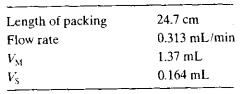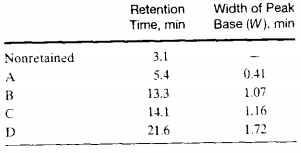Create an Account

Home / Questions / From the data in Problem 26 14 calculate for A B C and D a The retention factor b The di...

From the data in Problem 26 14 calculate for A B C and D a The retention factor b The distribution constant Problem 26 14 The following data are for a liquid chromatographic column

From the data in Problem 26-14, calculate for A, B, C. and D

(a) The retention factor.

(b) The distribution constant.

Problem 26-14

The following data are for a liquid chromatographic columnA chromatogram of a mixture of species A. B, C, and D provided the following data:Calculate

(a) The number of plates from each peak.

(b) The mean and the standard deviation for N.

(c) The plate height for the column.

Jun 23 2020 View more View LessSubscribe To Get Solution